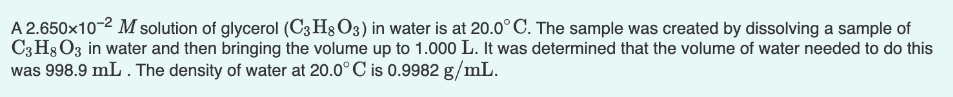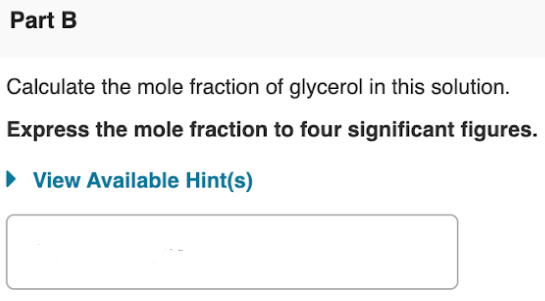# A 2.650x10^-2 M solution of glycerol (C3H8O3) in water is at 20.0°C. The sample was created by dissolving a sample of C3H8O3 in water and then bringing the volume up to 1.000 L. It was determined that the volume of water needed to do this was 998.9 mL. The density of water at 20.0°C is .09982 g/mL. Calculate the mole fraction of glycerol in this solution. Express the mole fraction to four significant figures.